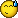90

please anybody kind me for Converting metatrader Indicators to AFL ,thanks a lot!!!!!!!!!nonono:

Files:6

sohel rana:
please anybody kind me for Converting metatrader Indicators to AFL ,thanks a lot!!!!!!!!!nonono:

I do not know what is AFL. ex4 attached. you are. I tried running.

Good luck.

Files:90

SIR AFL meaning ( amibroker fomula language)!6

sohel rana:
SIR AFL meaning ( amibroker fomula language)!

I do not know him. I'm sorry.1316

I think coding a indicator for AFL with help of metatrader indicator is much better than converting it from metatrader.90

Paul75, with all respect , i want to convert from mql4(metatrader cod) to AFL cod try to understan, thanks< here ex4 formate and mq4 formate, please please help me,

Files:90

Paul75

please sir help me help me ,maybe if u wish u will!9090

sohel rana:490

I NEED FOR AFL TO MQ4 CONVERT

//+------------------------------------ ------------------------------+

//| ZIG ZAG NON REPAINT.mq4 |

//+------------------------------------ ------------------------------+

#property indicator_chart_window

#property indicator_buffers 1

#property indicator_color1 Yellow

extern int Candle=100;

extern int Length=1;

double ExtMapBuffer1[];

int init()

{

SetIndexEmptyValue(0,0.0);

SetIndexStyle(0,DRAW_SECTION, 5,5);

SetIndexBuffer(0,ExtMapBuffer1);

return(0);

}

int deinit()

{

return(0);

}

int start()

{

int cnt,ticket,counted_bars=IndicatorCo unted();

int shift,Swing,Swing_n,uzl,i,zu,zd,mv;

double LL,HH,BH,BL,NH,NL;

double Uzel;

string text;

if (High> High) {Comment("SELL !!");}

else if (High< High) {Comment("BUY !!");}

Swing_n=0;Swing=0;uzl=0;

BH =High[Candle];BL=Low[Candle];zu=Candle;zd=Candle;

for (shift=Candle;shift>=0;shift--) {

LL=10000000;HH=-100000000;

for (i=shift+Length;i>=shift+1;i--) {

if (Low< LL) {LL=Low;}

if (High>HH) {HH=High;}

}

if (Low[shift]HH){

Swing=2;

if (Swing_n==1) {zu=shift+1;}

if (Swing_n==-1) {zd=shift+1;}

} else {

if (Low[shift]<LL) {Swing=-1;}

if (High[shift]>HH) {Swing=1;}

}

if (Swing!=Swing_n && Swing_n!=0) {

if (Swing==2) {

Swing=-Swing_n;BH = High[shift];BL = Low[shift];

}

uzl=uzl+1;

if (Swing==1) {

Uzel[uzl]=zd;

Uzel[uzl]=BL;

}

if (Swing==-1) {

Uzel[uzl]=zu;

Uzel[uzl]=BH;

}

BH = High[shift];

BL = Low[shift];

}

if (Swing==1) {

if (High[shift]>=BH) {BH=High[shift];zu=shift;}}

if (Swing==-1) {

if (Low[shift]<=BL) {BL=Low[shift]; zd=shift;}}

Swing_n=Swing;

}

for (i=1;i<=uzl;i++) {

mv=StrToInteger(DoubleToStr(Uzel,0));

ExtMapBuffer1[mv]=Uzel;

}

return(0);

}490

leenazen:
I NEED FOR MQ4 to AFL CONVERT //+------------------------------------ ------------------------------+ //| ZIG ZAG NON REPAINT.mq4 | //+------------------------------------ ------------------------------+ #property copyright "Copyright©2010" #property link "" #property indicator_chart_window #property indicator_buffers 1 #property indicator_color1 Yellow extern int Candle=100; extern int Length=1; double ExtMapBuffer1[]; int init() { SetIndexEmptyValue(0,0.0); SetIndexStyle(0,DRAW_SECTION, 5,5); SetIndexBuffer(0,ExtMapBuffer1); return(0); } int deinit() { return(0); } int start() { int cnt,ticket,counted_bars=IndicatorCo unted(); int shift,Swing,Swing_n,uzl,i,zu,zd,mv; double LL,HH,BH,BL,NH,NL; double Uzel; string text; if (High> High) {Comment("SELL !!");} else if (High=0;shift--) { LL=10000000;HH=-100000000; for (i=shift+Length;i>=shift+1;i--) { if (LowHH) {HH=High;} } if (Low[shift]HH){ Swing=2; if (Swing_n==1) {zu=shift+1;} if (Swing_n==-1) {zd=shift+1;} } else { if (Low[shift]HH) {Swing=1;} } if (Swing!=Swing_n && Swing_n!=0) { if (Swing==2) { Swing=-Swing_n;BH = High[shift];BL = Low[shift]; } uzl=uzl+1; if (Swing==1) { Uzel[uzl]=zd; Uzel[uzl]=BL; } if (Swing==-1) { Uzel[uzl]=zu; Uzel[uzl]=BH; } BH = High[shift]; BL = Low[shift]; } if (Swing==1) { if (High[shift]>=BH) {BH=High[shift];zu=shift;}} if (Swing==-1) { if (Low[shift]<=BL) {BL=Low[shift]; zd=shift;}} Swing_n=Swing; } for (i=1;i<=uzl;i++) { mv=StrToInteger(DoubleToStr(Uzel,0)); ExtMapBuffer1[mv]=Uzel; } return(0); }